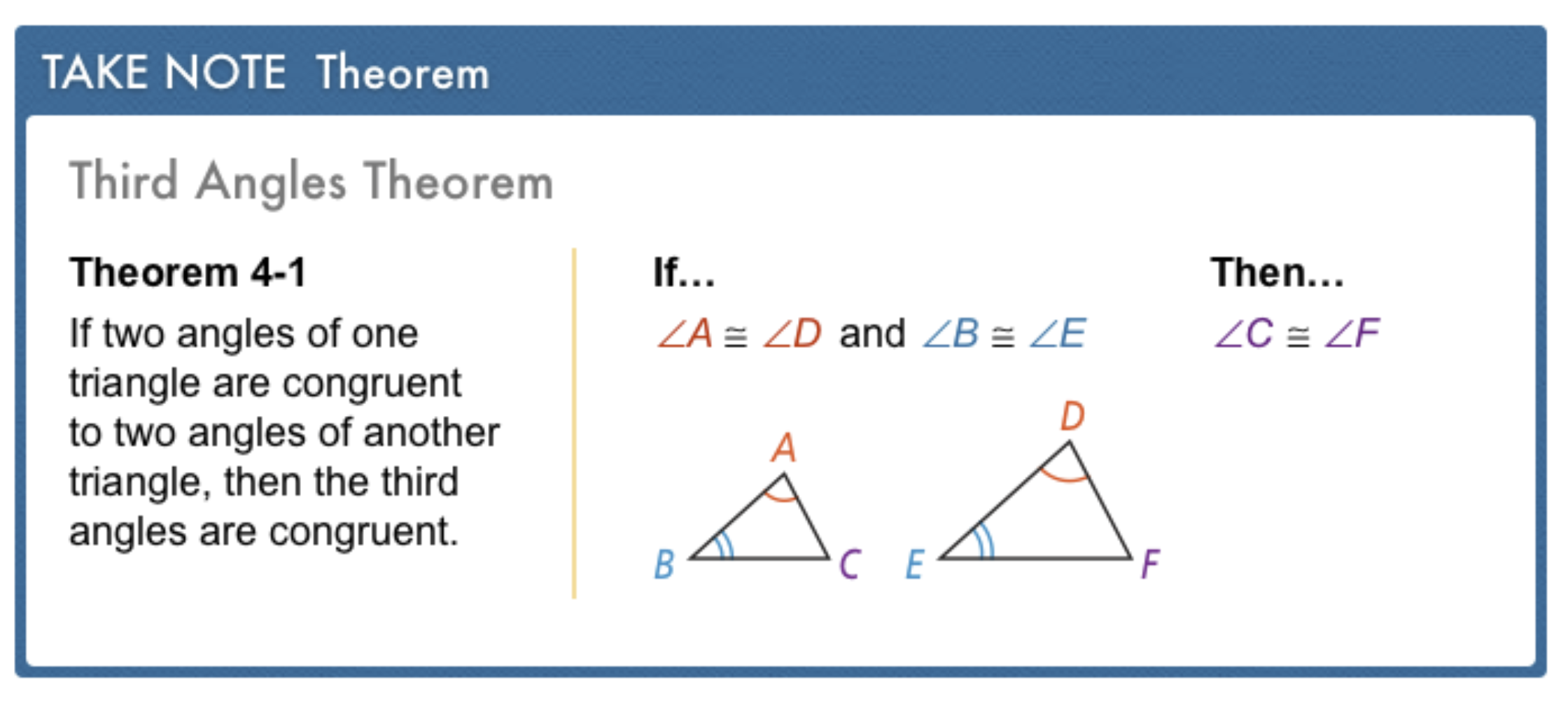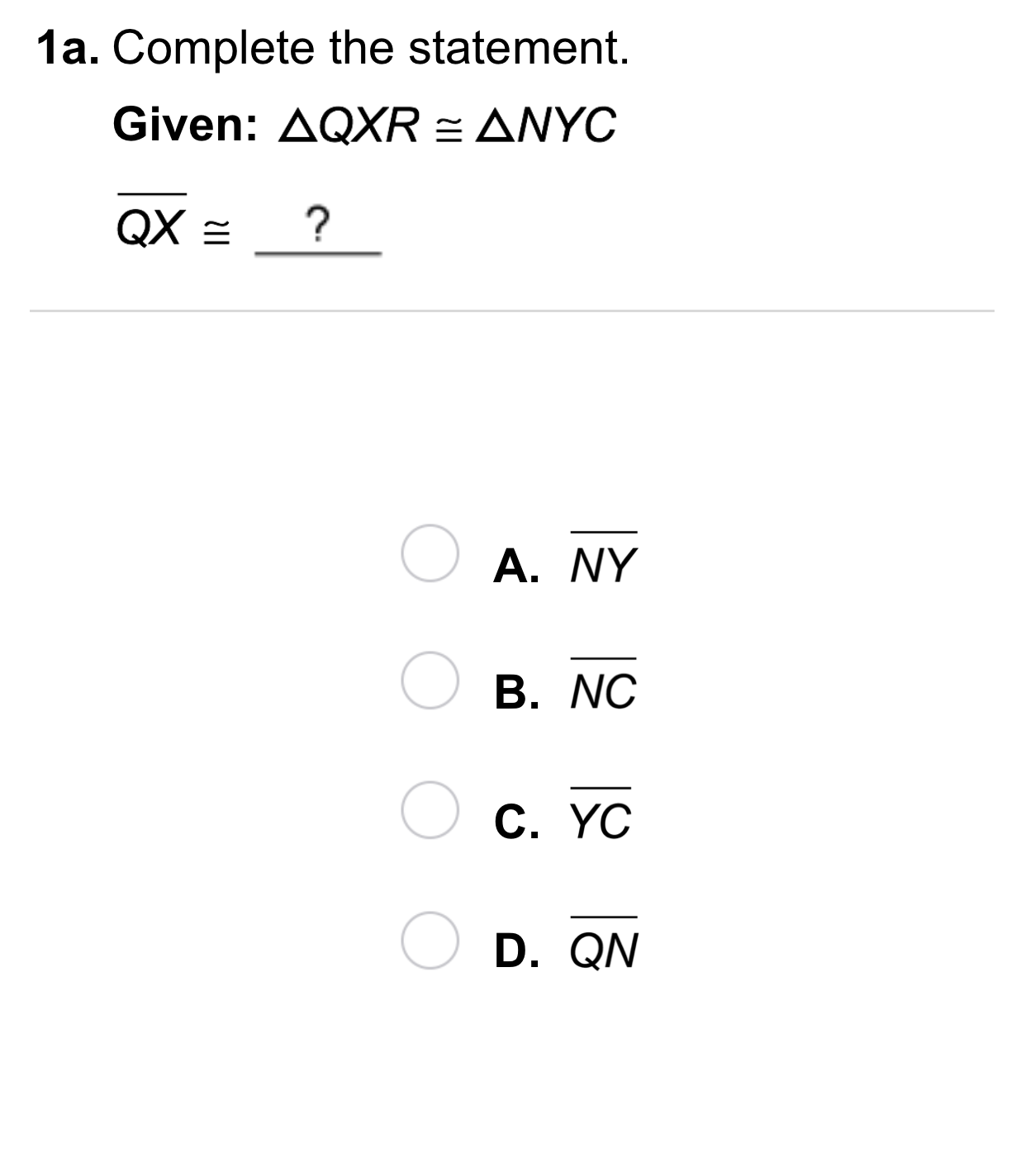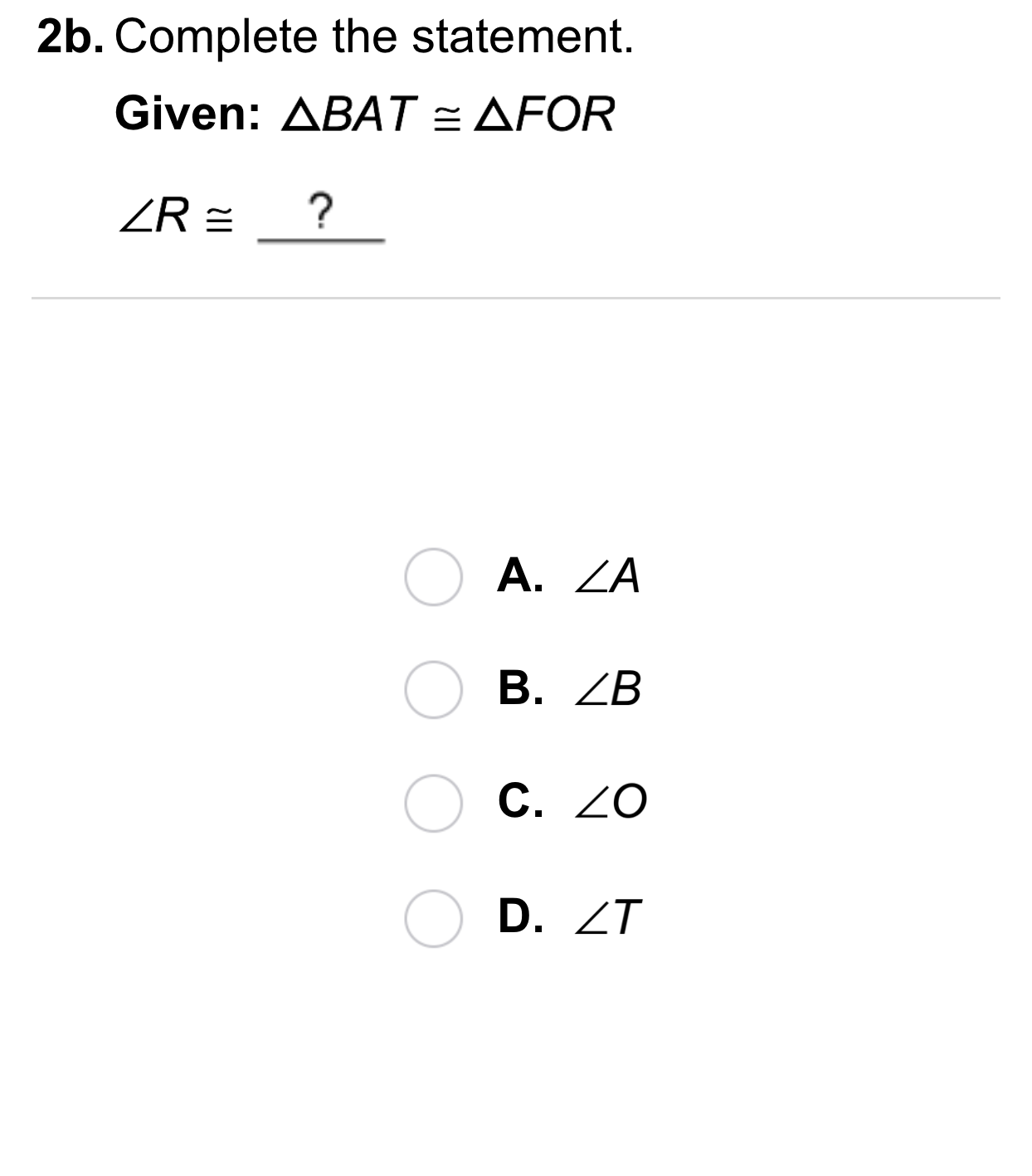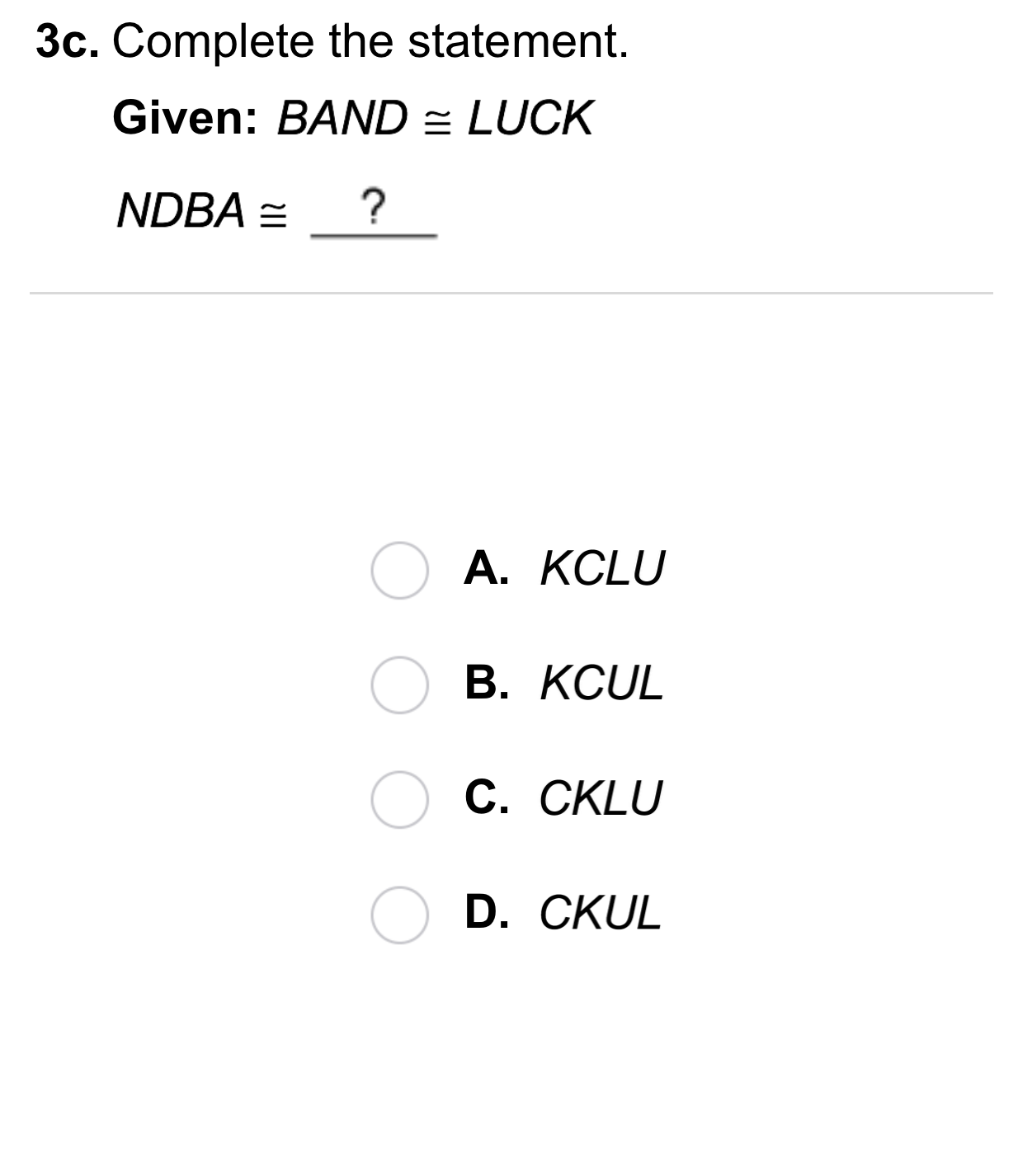Geometry 4-1 Congruent Figures
starstarstarstarstarstarstarstarstarstar
by Matthew Richardson
| 22 Questions
Note from the author:
A complete formative lesson with embedded slideshow, mini lecture screencasts, checks for understanding, practice items, mixed review, and reflection. I create these assignments to supplement each lesson of Pearson's Common Core Edition Algebra 1, Algebra 2, and Geometry courses. See also mathquest.net and twitter.com/mathquestEDU.
The outlined content above was added from outside of Formative.
The outlined content above was added from outside of Formative.1
1
10 pts
Solve It! You are working on a puzzle. You've almost finished, except for a few pieces of the sky. Match the puzzle piece numbers with the space letters to place the remaining pieces in the puzzle.
• 1
• 2
• 3
• A
• B
• CThe outlined content above was added from outside of Formative.2
2
10 pts
Problem 1 Got It?
Select all that apply.
A
B
C
D
The outlined content above was added from outside of Formative.
3
10 pts
Problem 2 Got It? Suppose the following:
What is m∠V? Enter only a number.
The outlined content above was added from outside of Formative.4
5
4
10 pts
Problem 3 Got It?

True
False
5
10 ptsThe outlined content above was added from outside of Formative.
6
40 pts
Problem 4 Got It? Complete the proof on the canvas. You may use any of the three styles learned: two-column, paragraph, or flow7
7
10 pts
A
B
C
D8
8
10 pts
A
B
C
D9
9
10 pts
A
B
C
D10
10
10 pts
A
B
C
D11
11
10 pts
A
B
C
D12
12
10 pts
A
B
C
D13
13
10 pts
A
B
C
D15
10 pts
Reasoning: If each angle in one triangle is congruent to its corresponding angle in another triangle, are the two triangles congruent? Explain.16
16
10 pts
Error Analysis: Walter sketched the diagram shown. He claims it shows that the two polygons are congruent. What information is missing to support his claim?17
10 pts
Review Lesson 3-8: Write an equation for a line perpendicular to the given line.

18
10 pts
Review Lesson 3-8: Write an equation for a line perpendicular to the given line.

19
10 pts
Review Lesson 1-7: Find the distance between the points. If necessary, round to the nearest tenth.
Enter only a number.20
10 pts
Vocabulary Review: Cross out the figure(s) that are NOT polygons.
21
10 pts
Use Your Vocabulary: Categorize each statement.
• Congruent angles have different measures.
• A prism and its net are congruent figures.
• The corresponding sides of congruent figures have the same measure.
• True
• False
22
10 pts
Reflection: Math Success